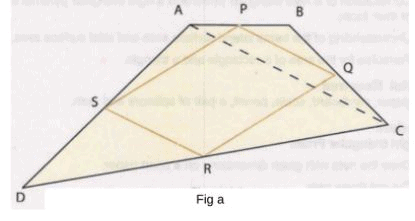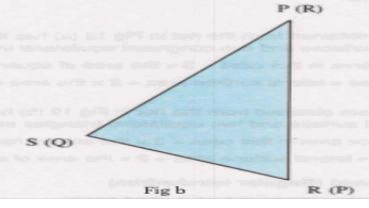# Procedure - To show that the figure obtained by joining the mid-points of consecutive sides, Math Class 9 Notes | EduRev

## Class 9 : Procedure - To show that the figure obtained by joining the mid-points of consecutive sides, Math Class 9 Notes | EduRev

The document Procedure - To show that the figure obtained by joining the mid-points of consecutive sides, Math Class 9 Notes | EduRev is a part of the Class 9 Course Mathematics (Maths) Class 9.
All you need of Class 9 at this link: Class 9

As performed in the real lab:

Materials required:

Colored papers, a pair of scissors, gum.

Procedure:

1. Cut off quadrilateral ABCD (Fig a) of paper with prescribed dimensions.
2. Mark the mid-points P,Q,R and S of the side AB,BC,CD,and AD respectively by folding the sides.
3. Cut off the quadrilateral PQRS.
4. Now cut the quadrilateral PQRS along the diagonal PR into two triangles PQR and PSR.
5. Superimpose the ▲ PSR on ▲ PQR such that PS falls on QR.(Fig b)
6. The ▲PQR covers the ▲PSR exactly.
7. Thus SP=QR and RS=PQ
8. Therefore, quadrilateral PQRS is a parallelogram.As performed in the simulator:

Procedure:

1. Form a quadrilateral ABCD by clicking on any 4 points in workbench area.
2. Mark mid-point of each line AB, BC, CD, AD as P, Q, R, S respectively.
3. Click and join midpoints of each side to form quadrilateral PQRS.
5. Click on point P and point R to draw diagonal PR.
6. Click on ▲PRQ and ▲ PRS respectively to fill them with distinct colors.
7. Click on "Cut Triangle" button to cut triangle PQR from quadrilateral PQRS.
8. ▲ PQR is draggable so you can drag it to any position in workbench area.
9. Rotate ▲ PQR using "Rotate Anti Clockwise" and "Rotate Clockwise" buttons.
10. Next step is to place triangle PQR over triangle PRS such that both of them overlap each other.

Observation:

• The ▲PQR overlaps ▲PSR.

• Thus SP=QR and RS=PQ.

• Therefore, quadrilateral PQRS is a parallelogram.

Offer running on EduRev: Apply code STAYHOME200 to get INR 200 off on our premium plan EduRev Infinity!

## Mathematics (Maths) Class 9

190 videos|233 docs|82 tests

,

,

,

,

,

,

,

,

,

,

,

,

,

,

,

,

,

,

,

,

,

,

,

,

;